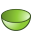# PB Card

This card can be used to generate a section of a parabolic reflector as shown in the figure on the card.

On the Construct tab, in the Surfaces group, click theParaboloid (PB) icon.

## Parameters:

S1
The centre of the paraboloid.
S2
A point perpendicular to the base plane and at any distance above the centre point.
S3
A point on the outer edge of the paraboloid, but on the base plane.
S4
A point in the plane S2–S1–S3, directly above S3 on the edge of the paraboloid.
Subtended angle $\phi$ (degrees)
The angle subtended by the arc of the parabolic reflector in degrees.
Maximum triangle edge length
Maximal edge length of the triangles along the outer edge of the arc in m (is scaled by the SF card). If this parameter is left empty, the value specified with the IP card is used.

The radius R of the paraboloid is derived from the distance between the points S1 and S3, as can be seen in the figure in the card. The height is determined by the distance between points S3 and S4. The focal point f is determined by:

(1) $f=\frac{{R}^{2}}{4h}$

## Example of PB card usage:

The parabolic reflector as shown can be generated with the PB card.